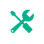# Absolute Value Calculator

testing Language
1. 1. testing Language testing Language
2. 2. testing Language testing Language
3. 3. orem Ipsum is simply dummy text of the printing and
LCM of
302
Finding LCM by Using Prime Factorization Method
Step 11
300 = 2 x 2 x 3 x 5 x 5
300 = 2 x 2
300 = 2 x 2 x 3
Step 2
300 = 22 x 3 x 52
30 = 2 x 3 x 5
Step 3
= 22 x 52 x 3
= 4 x 25 x 3
LCM(300, 30) = 300
Finding LCM by Using Division Table Method
Step 11
 2 30 300 2 15 150 3 15 75 5 5 25 5 1 5 1 1
Step 2
2 * 2 * 3 * 5 * 5
Step 3
LCM = 300
Finding LCM by List of Multiplies Method
Multiples of 300: 300 600 900
Multiples of 300: 300 600 900 600 900 600 900 600 900 600 900 600 900
LCM(300,30) = 300

## LCM Calculator

The online LCM calculator can find the least common multiple (factors) quickly than manual methods. Whether finding the LCM of two numbers or multiple numbers, this calculator can help you with just a single click.Related Tool Name

Thsi is lkajsdflkj